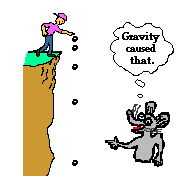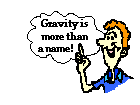Circular Motion and Satellite Motion - Lesson 3 - Universal Gravitation

# Gravity is More Than a Name

Nearly every child knows of the word gravity. Gravity is the name associated with the mishaps of the milkspilled from the breakfast table to the kitchen floor and the youngster who topples to the pavement as the grand finale of the first bicycle ride. Gravity is the name associated with the reason for "what goes up, must come down," whether it be the baseball hit in the neighborhood sandlot game or the child happily jumping on the backyard mini-trampoline. We all know of the word gravity - it is the thing that causes objects to fall to Earth. Yet the role of physics is to do more than to associate words with phenomenon. The role of physics is to explain phenomenon in terms of underlying principles. The goal is to explain phenomenon in terms of principles that are so universal that they are capable of explaining more than a single phenomenon but a wealth of phenomenon in a consistent manner. Thus, a student's conception of gravity must grow in sophistication to the point that it becomes more than a mere name associated with falling phenomenon. Gravity must be understood in terms of its cause, its source, and its far-reaching implications on the structure and the motion of the objects in the universe.

Certainly gravity is a force that exists between the Earth and the objects that are near it. As you stand upon the Earth, you experience this force. We have become accustomed to calling it the force of gravity and have even represented it by the symbol Fgrav. Most students of physics progress at least to this level of sophistication concerning the notion of gravity. This same force of gravity acts upon our bodies as we jump upwards from the Earth. As we rise upwards after our jump, the force of gravity slows us down. And as we fall back to Earth after reaching the peak of our motion, the force of gravity speeds us up. In this sense, the force gravity causes an acceleration of our bodies during this brief trip away from the earth's surface and back. In fact, many students of physics have become accustomed to referring to the actual acceleration of such an object as the acceleration of gravity. Not to be confused with the force of gravity (Fgrav), the acceleration of gravity (g) is the acceleration experienced by an object when the only force acting upon it is the force of gravity. On and near Earth's surface, the value for the acceleration of gravity is approximately 9.8 m/s/s. It is the same acceleration value for all objects, regardless of their mass (and assuming that the only significant force is gravity). Many students of physics progress this far in their understanding of the notion of gravity.

In Lesson 3, we will build on this understanding of gravitation, making an attempt to understand the nature of this force. Many questions will be asked: How and by whom was gravity discovered? What is the cause of this force that werefer to with the name of gravity? What variables affect the actual value of the force of gravity? Why does the force of gravity acting upon an object depend upon the location of the object relative to the Earth? How does gravity affect objects that are far beyond the surface of the Earth? How far-reaching is gravity's influence? And is the force of gravity that attracts my body to the Earth related to the force of gravity between the planets and the Sun? These are the questions that will be pursued. And if you can successfully answer them, then the sophistication of your understanding has extended beyond the point of merely associating the name "gravity" with falling phenomenon.

Next Section:
Jump To Next Lesson:

Follow Us